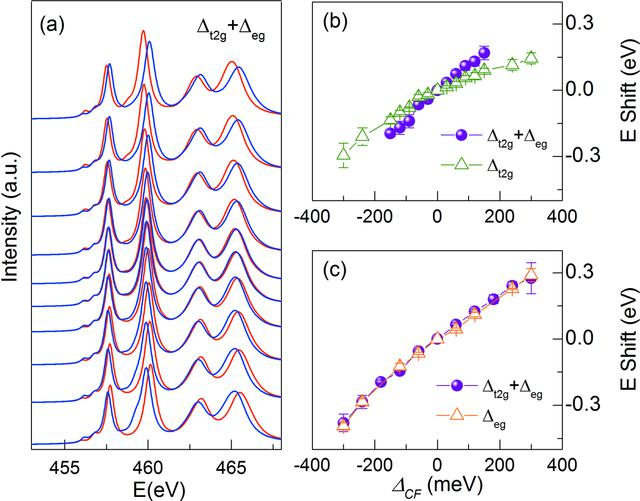disable zoom     view article Figure 6 (a) Calculated soft X-ray absorption spectra for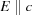and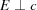photon polarizations at different crystal field splitting values, i.e.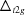of −150, −120, −90, −60, −30, 30, 60, 90, 120 and 150 meV from top to bottom spectra and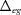=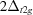. (b) Comparison of averaged energy shift of peak a1 and b1 for () and () polarizations between the spectra calculated considering bothandvalues and the spectra calculated considering only the splitting of t2g levels, i.e.. (c) Comparison of averaged energy shift of peak a2 and b2 between the spectra calculated for bothandvalues and the spectra calculated considering only.JOURNAL OFSYNCHROTRONRADIATION
ISSN: 1600-5775
Volume 25| Part 3| May 2018| Pages 777-784